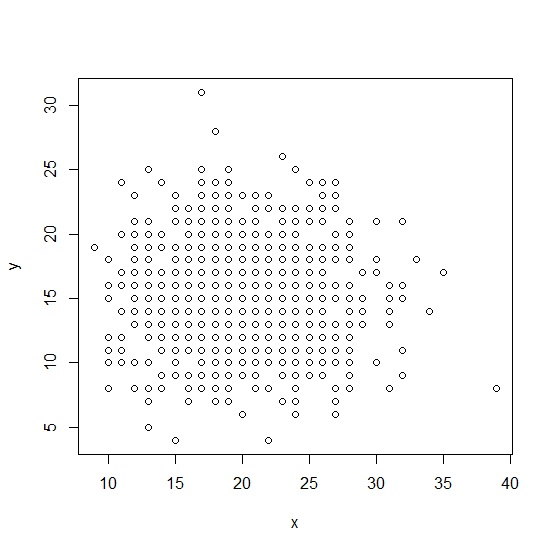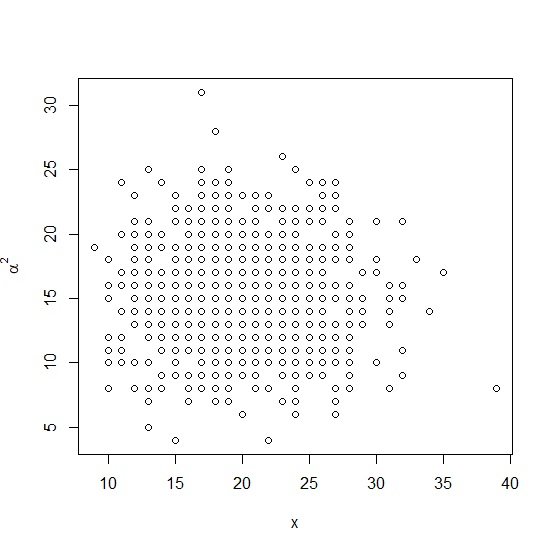# How to add a mathematical expression in axis label in a plot created by using plot function in R?

When we create a plot using plot function in R, the axes titles are either chosen by R automatically based on the vectors passed through the function or we can use ylab or xlab for particular axes. To add a mathematical expression in an axis label, we can use title function with expression function to define the mathematical expression.

Consider the below vectors and create scatterplot between the two −

## Example

set.seed(111)
x<-rpois(1000,20)
y<-rpois(1000,15)
plot(x,y)

## outputNow suppose, we want to remove y from the Y-axis and put alpha square then it can be done as shown below −

## Example

plot(x,y,ylab="")
title(ylab=expression(alpha^2))